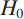×
Get Full Access to Statistics For Engineers And Scientists - 4 Edition - Chapter 6.12 - Problem 1e
Get Full Access to Statistics For Engineers And Scientists - 4 Edition - Chapter 6.12 - Problem 1e

×

# A hypothesis test is performed, and the P-value is 0.03.ISBN: 9780073401331 38

## Solution for problem 1E Chapter 6.12

Statistics for Engineers and Scientists | 4th Edition

• Textbook Solutions
• 2901 Step-by-step solutions solved by professors and subject experts
• Get 24/7 help from StudySoup virtual teaching assistantsStatistics for Engineers and Scientists | 4th Edition

4 5 1 422 Reviews
26
5
Problem 1E

Problem 1E

A hypothesis test is performed, and the P-value is 0.03. True or false:

a. H0 is rejected at the 5% level.

b. H0 is rejected at the 2% level.

c. H0 is not rejected at the 10% level.

Step-by-Step Solution:

Problem 1E

A hypothesis test is performed, and the P-value is 0.03. True or false:

a.is rejected at the 5% level.

b.is rejected at the 2% level.

c.is not rejected at the 10% level.

Step by Step Solution

Step 1 of 4

Given,

P-value = 0.03

We have to compare the P-value with each significance level to determine whether each statement is true or false.

Step 2 of 4

Step 3 of 4

##### ISBN: 9780073401331

The answer to “A hypothesis test is performed, and the P-value is 0.03. True or false:a. H0 is rejected at the 5% level.________________b. H0 is rejected at the 2% level.________________c. H0 is not rejected at the 10% level.” is broken down into a number of easy to follow steps, and 35 words. Since the solution to 1E from 6.12 chapter was answered, more than 329 students have viewed the full step-by-step answer. This full solution covers the following key subjects: level, rejected, performed, False, hypothesis. This expansive textbook survival guide covers 153 chapters, and 2440 solutions. Statistics for Engineers and Scientists was written by and is associated to the ISBN: 9780073401331. The full step-by-step solution to problem: 1E from chapter: 6.12 was answered by , our top Statistics solution expert on 06/28/17, 11:15AM. This textbook survival guide was created for the textbook: Statistics for Engineers and Scientists , edition: 4.

Unlock Textbook Solution